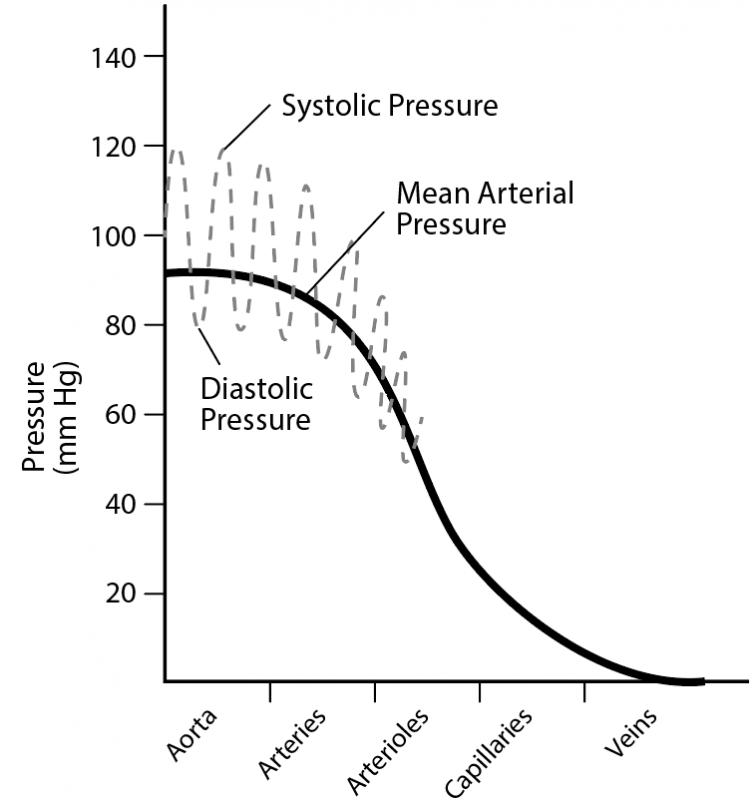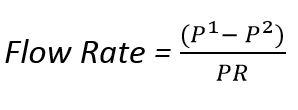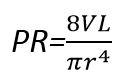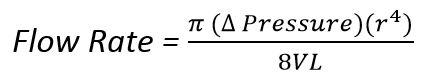2.6.1

# Blood Pressure Explained

Blood pressure is tightly controlled. Homeostasis mechanisms assure that blood pressure does not get too low or life sustaining organs like the brain can be in danger of permanent damage. You may hear the term Pulse Pressure. Pulse pressure is the pressure difference between the peak systolic and diastolic pressures. For example, if the systolic pressure is 120 mmHg and the diastolic pressure is 80 mmHg, then the pulse pressure is 40 mmHg.

Another term to understand is Mean Arterial Pressure (MAP). In Module 1 you learned that MAP was equal to the cardiac output multiplied by the total peripheral resistance (PR). Another way in which MAP can be calculated is by taking a weighted average of systolic and diastolic blood pressure. "Weighted average means that diastolic pressure has more influence on the "average" pressure for arteries because diastole lasts longer than systole. MAP is calculated using the formula: MAP=Diastolic Pressure + 1/3 (Systolic Pressure - Diastolic Pressure).

The image below shows the mean arterial pressure as a solid line dropping from about 100 in the aorta to 0 in the veins.MAP = CO X PR

CO = HR X SV

PR ∝ L*V/r4

MAP = Mean Arterial Pressure; CO = Cardiac Output; PR=Peripheral Resistance;HR= Heart Rate; SV=Stroke Volume; L = Length; V=Viscosity; r=radius of a blood vessel

The concept of "PR ∝ L*V/r4" Comes from work done by a French physicist named Jean Léonard Marie Poiseuille (1797 - 1869). Poiseuille studied flow rates of fluid in pipes. His principles apply relatively well to human blood vessels.

Two equations help define his work on flowrate.This equation suggests that more fluid will flow through a vessel if the pressure difference from the start (P1) and end (P2) of the vessel is high (i.e. a high blood pressure) and the peripheral resistance to blood flow (PR) is low. This second equation defines the factors that determine peripheral resistance.When these two equations are combined we get an equation that defines "Poiseuille's Law".According to Poiseuille's Law, vasodilation would result in a very significant increase in blood flow through a vessel. Also, vasoconstriction would cause blood flow to drop (notice "r" to the 4th power). The muscular arteries and arterioles of the human circulatory system can change their radius remarkably with vasodilation and vasoconstriction.

The blood pressure in the large arteries is what is being determined when a blood pressure is taken. The equations above help us visualize the variables that can regulate and change blood pressure. Notice that the peripheral resistance is listed as being "proportional" (use of the proportional symbol ‘∝’) to blood vessel length and blood viscosity but inversely proportional to radius of the blood vessel raised to the 4th power! The proportional sign (∝) is used because the actual equation for peripheral resistance uses some constants not shown here. We do not intend to actually calculate peripheral resistances, but we do want to see how the variables relate to each other.

Consider the following example:

During exercise, muscles pump and return more blood to the heart. The heart beats with stronger contractions to evacuate the extra blood returning to the heart. This makes stroke volume go up. During exercise, heart rate also increases. If HR and SV increase, then CO increases and the equations above show us that an increase in CO would increase MAP. An increase in MAP helps maintain adequate blood flow to working muscles during exercise. A discussion of blood pressure is really a discussion of how the body controls the variables in the equations mentioned to keep blood pressure in homeostatic ranges.